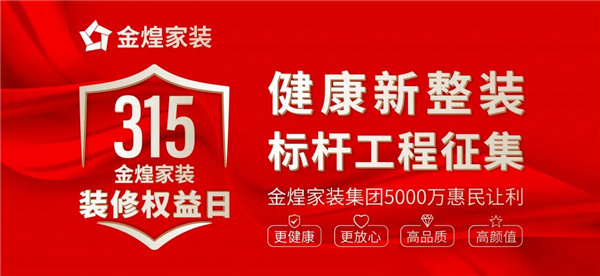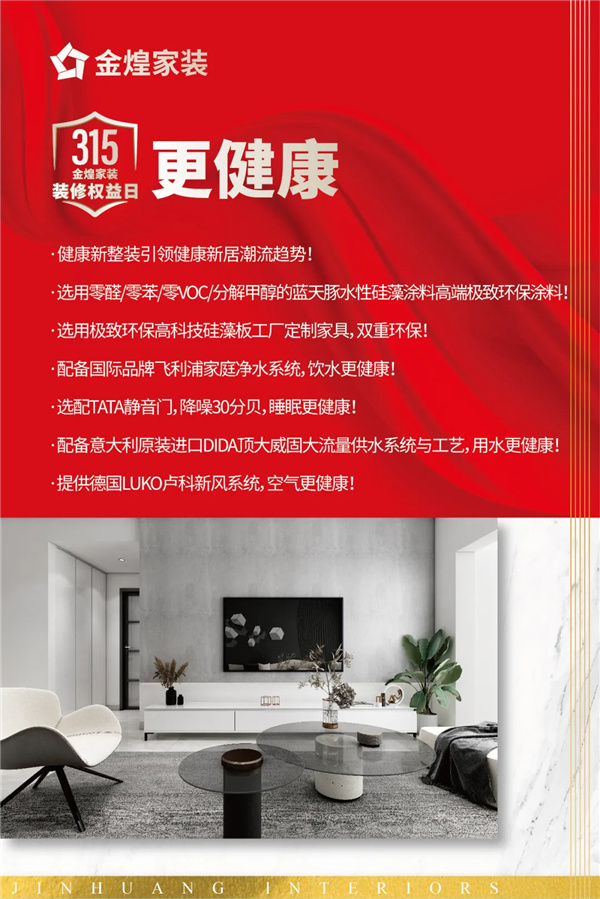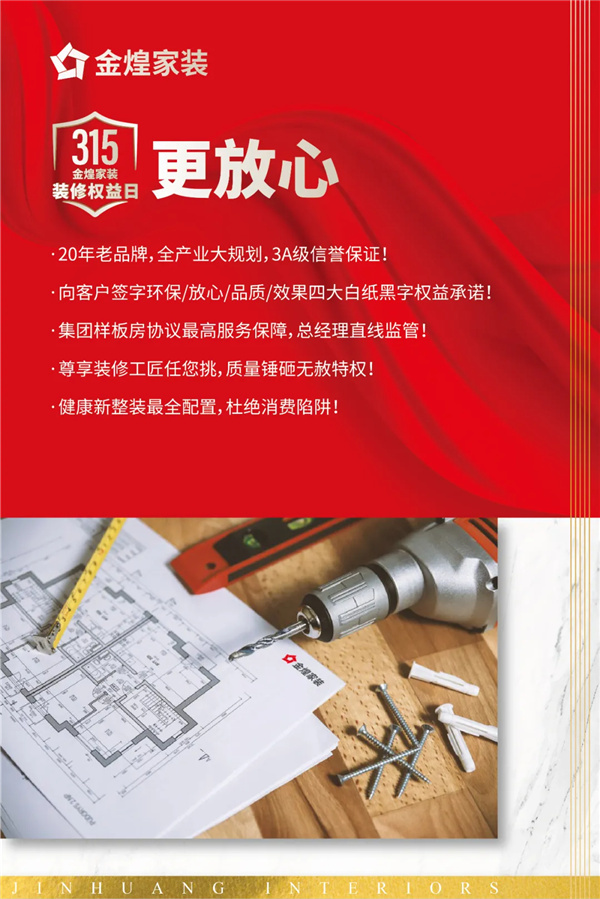|

# 315装修权益日,金煌健康新整装标杆工程征集,品质家装更放心

一年一度的“3.15国际消费者权益日”即将到来，关注消费者权益保护的话题，又重回大众视线。家装行业的特性是服务周期长、过程接触点多，对于消费者权益的保护和满意度要求更高。金煌家装作为行业标杆企业，重磅推出“3.15金煌家装装修权益日”活动，以此做出表率，重视装修业主权益的保护，珍视装修业主的满意度，将20年的行业积淀，从信誉、质量、环保、健康四个方面，回馈到广大装修业主的“装房大事”上。当前家装行业乱象很多：低价营销恶意增项、虚价宣传、环保不达标、售后推诿不负责……造成行业信任低、客户满意度低。这些问题让家装业主无不担忧着！家庭装修是幸福生活的一项重要元素，品质的好坏影响着人们生活的幸福感。作为湖南3A信誉装企的金煌家装，通过“16不限、8全免、0增项”、“免费环保检测”等具体措施标准，打破行业潜规则，是我们引领行业健康、良性发展的重要责任。金煌家装始终坚持服务以消费者为中心，一路走来收获百万业主口碑褒奖与力荐。在“3.15国际消费者权益日”之际，金煌家装再次倡导与履行社会责任，重磅推出征集健康标杆样板工程，同时推出四大放心承诺，对家装质量做出保证，全面打消您的装修顾虑。此次活动推出放心承诺的同时，金煌家装全省15家门店还同步推出5000万惠民让利补贴！以此作为金煌家装服务提质的重大举措，以更健康、更放心、高品质、高颜值的家装服务，彻底颠覆传统家装的诚信不足和低价套餐品质低下的诟病，为广大业主筑就健康美好家。

更健康 Healthier

墙面装修时所采用的蓝天豚水性硅藻涂料本身不含甲醛，还能吸附分解甲醛、苯等有毒分子，硅藻土“分子筛”结构具有物理吸附、分解能力；能调节空气湿度，防止霉菌滋生，是居家墙体装饰的优选产品。

金煌率先应用国际领先零醛硅藻板定制家具，其设计造型百变、防火阻燃、防潮防虫等特点，突破了传统定制家具的不环保、易潮易霉易变形等缺陷，从健康、耐用、安全、美观四个方面革新定制家具的新高度。

根据业主的实际需求，配备国际品牌飞利浦家庭净水系统，拥有多重过滤技术，层层过滤捍卫家庭水健康；创新水路连接方案，密封性更强更安全，饮水更健康。

配备意大利原装进口DIDA顶大威固大流量供水系统与工艺，管材内层纯PPR透明层，解决水垢附着，自洁卫生，无任何色素配方，绿色无毒。杜绝用水隐患，用水更健康！

提供业主最优化的睡眠条件，配备TATA静音门，独创的45°斜角，另外融入新型软磁吸门设计，使TATA木门能够做到最小隔音30分贝，睡眠更健康。

配备德国LUKO卢科新风系统，能有效控制室内温度处于20～26℃，相对湿度40～65%，CO?浓度1000PPM以下，热回收效率75%以上，并显著降低室内PM2.5浓度，全天候保证室内空气温湿度环境和空气清新。更放心 More assured

金煌家装作为湖南装饰行业龙头企业，连续多年零投诉，被评定为信誉AAA级企业，历年多次受省市领导视察与嘉奖。

主动签定《环保承诺书》、《质量承诺书》、《效果承诺书》、《价格承诺书》，白纸黑字才是真正的承诺。

签定集团样板房协议最高服务保障，总经理直线监管，为业主的健康生活品质保驾护航！

尊享装修工匠任您挑，装修过程中质量不合格、环保不达标砸掉重做，费用由金煌家装承担，坚持为客户带来独一无二的装修体验。

健康新整装最全配置，通过与家居生活息息相关的空气、水、睡眠、温度等9大硬核健康产品体系，全方位满足人们对健康人居环境的需求，杜绝消费陷阱！高品质 High-quality

甄选一线基材主材品牌，与全球200余家国际国内知名品牌达成战略联盟。

与全国20多家著名地产商形成良好合作关系，其庞大的采购量是别的装饰公司和建材公司比拟不了的，采购量大，工厂批量定制生产，能在工厂价的基础上再省30%。

32大标准精装工艺，6大施工规范，极致打磨金钻工艺，5级质检保障施工品质，全方位严格保障工程质量，标准化施工，精严品质实时查验。

施工班组军事化管理，金煌装修工匠入职都要经过培训考核合格后才能上岗，并且在施工操作中严格执行每一项工艺标准，为每一位业主提供金牌工匠工程服务。高颜值 Good-looking

简约、田园、中式、欧式、美式等8大风格家具任选，由金煌专业室内设计师亲自搭配，省却装修新手们的搭配烦恼。

大板全抛釉瓷砖，有颜值有质量，多种色彩可供选择，满足个性化装修的需求。

金煌四人设计团队共同设计，个性化原创设计，根据业主需求量身定制设计方案，设计0复制，一户一设计，真正做到家家量身定制，户户与众不同。

为客户提供全景设计方案，导入客餐厅全景动态效果，提前预见新家。每一位家装业主都是上帝！当业主将钥匙交付给我们的那刻，我们深感责任重大。秉承着“做良心家装，做有幸福感的家装”、“装修不环保，等于谋财害命”的服务初心，最早推出“全包整装”、提出“家装标准化”、打造“健康新整装”。让装修不再成为“烦心事”、“麻烦事”，而是变成一种美好的过程体验，您的眼睛和满意度就是我们的最高标准。

`声明：本文由入驻焦点开放平台的作者撰写，除焦点官方账号外，观点仅代表作者本人，不代表焦点立场错误信息举报电话： 400-099-0099，邮箱：jubao@vip.sohu.com，或点此进行意见反馈，或点此进行举报投诉。`A B C D E F G H J K L M N P Q R S T W X Y Z
A - B - C - D - E
• A
• 鞍山
• 安庆
• 安阳
• 安顺
• 安康
• 澳门
• B
• 北京
• 保定
• 包头
• 巴彦淖尔
• 本溪
• 蚌埠
• 亳州
• 滨州
• 北海
• 百色
• 巴中
• 毕节
• 保山
• 宝鸡
• 白银
• 巴州
• C
• 承德
• 沧州
• 长治
• 赤峰
• 朝阳
• 长春
• 常州
• 滁州
• 池州
• 长沙
• 常德
• 郴州
• 潮州
• 崇左
• 重庆
• 成都
• 楚雄
• 昌都
• 慈溪
• 常熟
• D
• 大同
• 大连
• 丹东
• 大庆
• 东营
• 德州
• 东莞
• 德阳
• 达州
• 大理
• 德宏
• 定西
• 儋州
• 东平
• E
• 鄂尔多斯
• 鄂州
• 恩施
F - G - H - I - J
• F
• 抚顺
• 阜新
• 阜阳
• 福州
• 抚州
• 佛山
• 防城港
• G
• 赣州
• 广州
• 桂林
• 贵港
• 广元
• 广安
• 贵阳
• 固原
• H
• 邯郸
• 衡水
• 呼和浩特
• 呼伦贝尔
• 葫芦岛
• 哈尔滨
• 黑河
• 淮安
• 杭州
• 湖州
• 合肥
• 淮南
• 淮北
• 黄山
• 菏泽
• 鹤壁
• 黄石
• 黄冈
• 衡阳
• 怀化
• 惠州
• 河源
• 贺州
• 河池
• 海口
• 红河
• 汉中
• 海东
• 怀来
• I
• J
• 晋中
• 锦州
• 吉林
• 鸡西
• 佳木斯
• 嘉兴
• 金华
• 景德镇
• 九江
• 吉安
• 济南
• 济宁
• 焦作
• 荆门
• 荆州
• 江门
• 揭阳
• 金昌
• 酒泉
• 嘉峪关
K - L - M - N - P
• K
• 开封
• 昆明
• 昆山
• L
• 廊坊
• 临汾
• 辽阳
• 连云港
• 丽水
• 六安
• 龙岩
• 莱芜
• 临沂
• 聊城
• 洛阳
• 漯河
• 娄底
• 柳州
• 来宾
• 泸州
• 乐山
• 六盘水
• 丽江
• 临沧
• 拉萨
• 林芝
• 兰州
• 陇南
• M
• 牡丹江
• 马鞍山
• 茂名
• 梅州
• 绵阳
• 眉山
• N
• 南京
• 南通
• 宁波
• 南平
• 宁德
• 南昌
• 南阳
• 南宁
• 内江
• 南充
• P
• 盘锦
• 莆田
• 平顶山
• 濮阳
• 攀枝花
• 普洱
• 平凉
Q - R - S - T - W
• Q
• 秦皇岛
• 齐齐哈尔
• 衢州
• 泉州
• 青岛
• 清远
• 钦州
• 黔南
• 曲靖
• 庆阳
• R
• 日照
• 日喀则
• S
• 石家庄
• 沈阳
• 双鸭山
• 绥化
• 上海
• 苏州
• 宿迁
• 绍兴
• 宿州
• 三明
• 上饶
• 三门峡
• 商丘
• 十堰
• 随州
• 邵阳
• 韶关
• 深圳
• 汕头
• 汕尾
• 三亚
• 三沙
• 遂宁
• 山南
• 商洛
• 石嘴山
• T
• 天津
• 唐山
• 太原
• 通辽
• 铁岭
• 泰州
• 台州
• 铜陵
• 泰安
• 铜仁
• 铜川
• 天水
• 天门
• W
• 乌海
• 乌兰察布
• 无锡
• 温州
• 芜湖
• 潍坊
• 威海
• 武汉
• 梧州
• 渭南
• 武威
• 吴忠
• 乌鲁木齐
X - Y - Z
• X
• 邢台
• 徐州
• 宣城
• 厦门
• 新乡
• 许昌
• 信阳
• 襄阳
• 孝感
• 咸宁
• 湘潭
• 湘西
• 西双版纳
• 西安
• 咸阳
• 西宁
• 仙桃
• 西昌
• Y
• 运城
• 营口
• 盐城
• 扬州
• 鹰潭
• 宜春
• 烟台
• 宜昌
• 岳阳
• 益阳
• 永州
• 阳江
• 云浮
• 玉林
• 宜宾
• 雅安
• 玉溪
• 延安
• 榆林
• 银川
• Z
• 张家口
• 镇江
• 舟山
• 漳州
• 淄博
• 枣庄
• 郑州
• 周口
• 驻马店
• 株洲
• 张家界
• 珠海
• 湛江
• 肇庆
• 中山
• 自贡
• 资阳
• 遵义
• 昭通
• 张掖
• 中卫

1室1厅1厨1卫1阳台

1
2
3
4
5

0
1
2

1

1

0
1
2
3报名成功，资料已提交审核A B C D E F G H J K L M N P Q R S T W X Y Z
A - B - C - D - E
• A
• 鞍山
• 安庆
• 安阳
• 安顺
• 安康
• 澳门
• B
• 北京
• 保定
• 包头
• 巴彦淖尔
• 本溪
• 蚌埠
• 亳州
• 滨州
• 北海
• 百色
• 巴中
• 毕节
• 保山
• 宝鸡
• 白银
• 巴州
• C
• 承德
• 沧州
• 长治
• 赤峰
• 朝阳
• 长春
• 常州
• 滁州
• 池州
• 长沙
• 常德
• 郴州
• 潮州
• 崇左
• 重庆
• 成都
• 楚雄
• 昌都
• 慈溪
• 常熟
• D
• 大同
• 大连
• 丹东
• 大庆
• 东营
• 德州
• 东莞
• 德阳
• 达州
• 大理
• 德宏
• 定西
• 儋州
• 东平
• E
• 鄂尔多斯
• 鄂州
• 恩施
F - G - H - I - J
• F
• 抚顺
• 阜新
• 阜阳
• 福州
• 抚州
• 佛山
• 防城港
• G
• 赣州
• 广州
• 桂林
• 贵港
• 广元
• 广安
• 贵阳
• 固原
• H
• 邯郸
• 衡水
• 呼和浩特
• 呼伦贝尔
• 葫芦岛
• 哈尔滨
• 黑河
• 淮安
• 杭州
• 湖州
• 合肥
• 淮南
• 淮北
• 黄山
• 菏泽
• 鹤壁
• 黄石
• 黄冈
• 衡阳
• 怀化
• 惠州
• 河源
• 贺州
• 河池
• 海口
• 红河
• 汉中
• 海东
• 怀来
• I
• J
• 晋中
• 锦州
• 吉林
• 鸡西
• 佳木斯
• 嘉兴
• 金华
• 景德镇
• 九江
• 吉安
• 济南
• 济宁
• 焦作
• 荆门
• 荆州
• 江门
• 揭阳
• 金昌
• 酒泉
• 嘉峪关
K - L - M - N - P
• K
• 开封
• 昆明
• 昆山
• L
• 廊坊
• 临汾
• 辽阳
• 连云港
• 丽水
• 六安
• 龙岩
• 莱芜
• 临沂
• 聊城
• 洛阳
• 漯河
• 娄底
• 柳州
• 来宾
• 泸州
• 乐山
• 六盘水
• 丽江
• 临沧
• 拉萨
• 林芝
• 兰州
• 陇南
• M
• 牡丹江
• 马鞍山
• 茂名
• 梅州
• 绵阳
• 眉山
• N
• 南京
• 南通
• 宁波
• 南平
• 宁德
• 南昌
• 南阳
• 南宁
• 内江
• 南充
• P
• 盘锦
• 莆田
• 平顶山
• 濮阳
• 攀枝花
• 普洱
• 平凉
Q - R - S - T - W
• Q
• 秦皇岛
• 齐齐哈尔
• 衢州
• 泉州
• 青岛
• 清远
• 钦州
• 黔南
• 曲靖
• 庆阳
• R
• 日照
• 日喀则
• S
• 石家庄
• 沈阳
• 双鸭山
• 绥化
• 上海
• 苏州
• 宿迁
• 绍兴
• 宿州
• 三明
• 上饶
• 三门峡
• 商丘
• 十堰
• 随州
• 邵阳
• 韶关
• 深圳
• 汕头
• 汕尾
• 三亚
• 三沙
• 遂宁
• 山南
• 商洛
• 石嘴山
• T
• 天津
• 唐山
• 太原
• 通辽
• 铁岭
• 泰州
• 台州
• 铜陵
• 泰安
• 铜仁
• 铜川
• 天水
• 天门
• W
• 乌海
• 乌兰察布
• 无锡
• 温州
• 芜湖
• 潍坊
• 威海
• 武汉
• 梧州
• 渭南
• 武威
• 吴忠
• 乌鲁木齐
X - Y - Z
• X
• 邢台
• 徐州
• 宣城
• 厦门
• 新乡
• 许昌
• 信阳
• 襄阳
• 孝感
• 咸宁
• 湘潭
• 湘西
• 西双版纳
• 西安
• 咸阳
• 西宁
• 仙桃
• 西昌
• Y
• 运城
• 营口
• 盐城
• 扬州
• 鹰潭
• 宜春
• 烟台
• 宜昌
• 岳阳
• 益阳
• 永州
• 阳江
• 云浮
• 玉林
• 宜宾
• 雅安
• 玉溪
• 延安
• 榆林
• 银川
• Z
• 张家口
• 镇江
• 舟山
• 漳州
• 淄博
• 枣庄
• 郑州
• 周口
• 驻马店
• 株洲
• 张家界
• 珠海
• 湛江
• 肇庆
• 中山
• 自贡
• 资阳
• 遵义
• 昭通
• 张掖
• 中卫• 手机• 分享
• 设计
免费设计
• 计算器
装修计算器
• 入驻
合作入驻
• 联系
联系我们
• 置顶
返回顶部